Next: Track Fitting in r-z Up: Track Fitting in r- Previous: Inclusion of Multiple Scattering

## Track Fitting under the Influence of Multiple Scattering

When the track is affected by multiple scattering during its coordinate measurements the error matrix (E0 in Eq.3.2.15) should take that into account. To see how to implement multiple scattering, let us start with the modification of the track equation (Eq.3.2.7):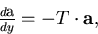where we have set (x0,y0) = (0,0) and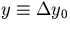.Multiple scattering modifies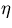by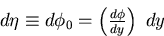(58)
which necessitates the modification of the track equationto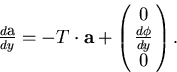(59)
This means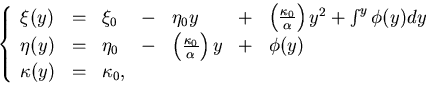(60)
where the suffix attached to the track track parameters remines us that they are the track parameters at the starting point before any multiple scattering. In the pivot convention taken here (x0,y0) = (0,0),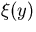is the x-location of the track. Therefore, the probability of observing (n+1) hit points,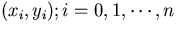and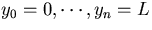, is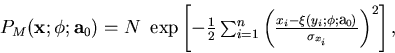(61)
where N is a normalization factor and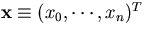.We must, however, take into account the probability of obtaining the multiple scattering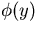: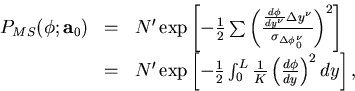(62)
where use has been made of Eq.3.2.11. Since we do not know, we should integrate the probability over: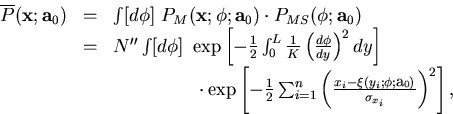(63)
which now gives the probability of observing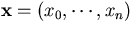when the track has the track parameter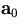at y=0.

Now, we fit the (n+1) hits to our track model (Eq.3.1.1) by minimizing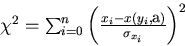(64)
where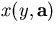is given by Eq.3.1.1 with x0=0. Since this problem is linear, we can easily obtain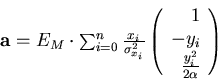(65)
with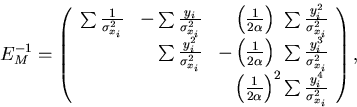(66)
where EM-1 is the inverse of the error matrix due to corrdinate measurement errors, for which only the upper triangle is explicitly shown. Of course, the true error matrix is subsect to the correction for multiple scattering. Let us now derive the true error matrix below. We first note that the displacement of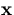induces the displacement of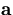through Eq.3.2.34: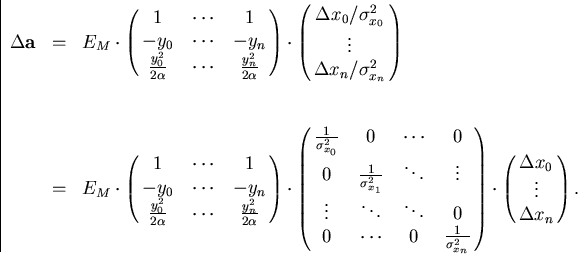(67)

Defining A and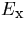by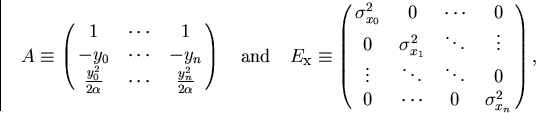(68)
we can rewrite Eq.3.2.36 as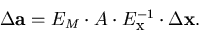(69)
This equation implies the full error matrix including multiple scattering to be given by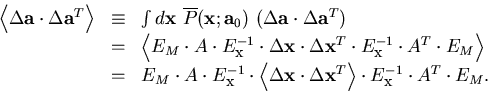(70)
Notice that in the absence of multiple scattering, we have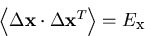and, therefore,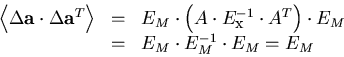as it should be, where in the last line, we have used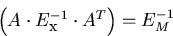(71)

Our problem is now reduces to how to evaluate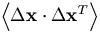when the multiple scattering correlates, for instance,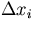to.In order to calculate the correlation matrix, we first note that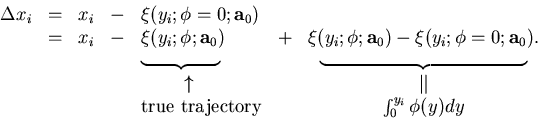(72)
Substituting this in Eq.3.2.32, we obtain the following formula for the probability of getting the measured points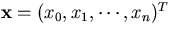for a track whose track parameter vector at y=y0 is: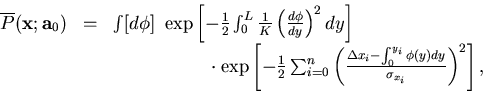(73)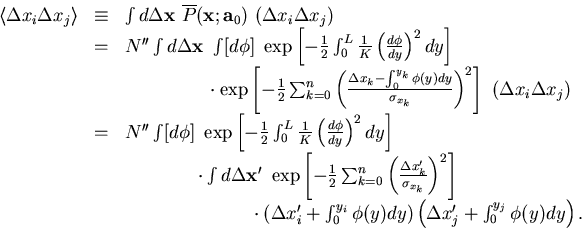The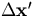integral is readily performed to yield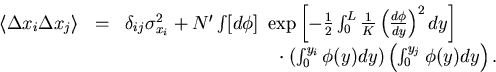(74)
The second term, which was induced by multiple scattering, has a familiar path integral form in field theories. After some straightforward manipulations such as to divide the path into small segments and to replace integrals by summentions and differentiations by differences, we arrive at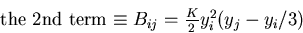(75)
for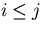which is enough since B is apparently symmetric. Combining Eqs.3.2.39, 3.2.46, and 3.2.47, we obtain the full error matrix as the sum of the part due to coordinate measurement errors EM and the part due to the multiple scattering in the tracking volume EMS: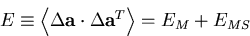(76)
with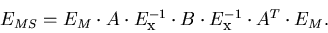(77)
The calculation of EMS is tedious but doable. We will, however, restrict ourselves to the case where the (n+1) samples are equally spaced in y and measured with an equal accuracy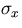.We fill further assume that n is large, since the final expression otherwise becomes too complicated to show here. The large n limit then gives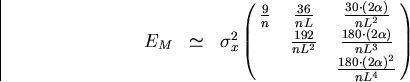(78)
and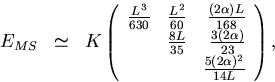(79)
which, for instance, result in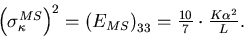The error on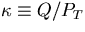due to multiple scattering is thus obtained to be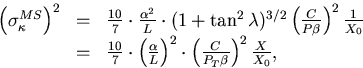where use has been made of Eq.3.2.11 and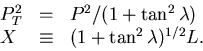The X is the material thickness through which the track passes. To see the dependence on the magnetic field explicitly, we introduce a new constant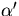defined by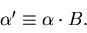When we assume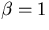and |Q|=1 (unit charge), we finally get a familiar result: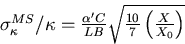(80)
with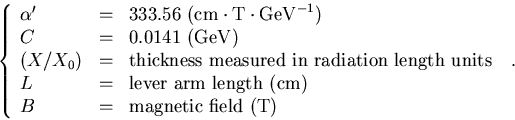(81)Next: Track Fitting in r-z Up: Track Fitting in r- Previous: Inclusion of Multiple Scattering
Keisuke Fujii
12/4/1998